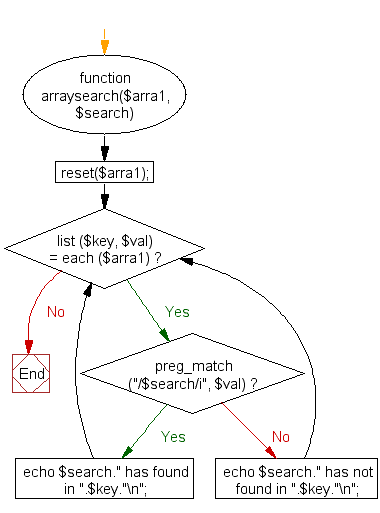﻿ PHP Array Exercise: Search a specified value within the values of an associative array - w3resource# PHP Array Exercises : Search a specified value within the values of an associative array

## PHP Array: Exercise-33 with Solution

Write a PHP function to search a specified value within the values of an associative array.

Sample Solution:

PHP Code:

``````<?php
function arraysearch(\$arra1, \$search)
{
reset(\$arra1);
while (list (\$key, \$val) = each (\$arra1))
{
if (preg_match ("/\$search/i", \$val))
{
echo \$search." has found in ".\$key."\n";
}
else
{
}
}
}
\$exercises = array("part1"=>"PHP array", "part2"=>"PHP String", "part3"=>"PHP Math");
arraysearch(\$exercises, "Math");
?>
```
```

Sample Output:

```Math has not found in part1
Math has found in part3
```

Flowchart:PHP Code Editor:

What is the difficulty level of this exercise?

﻿

## PHP: Tips of the Day

PHP: Extract numbers from a string

```\$str = 'In My Cart : 11 12 items';
preg_match_all('!\d+!', \$str, \$matches);
print_r(\$matches);
```